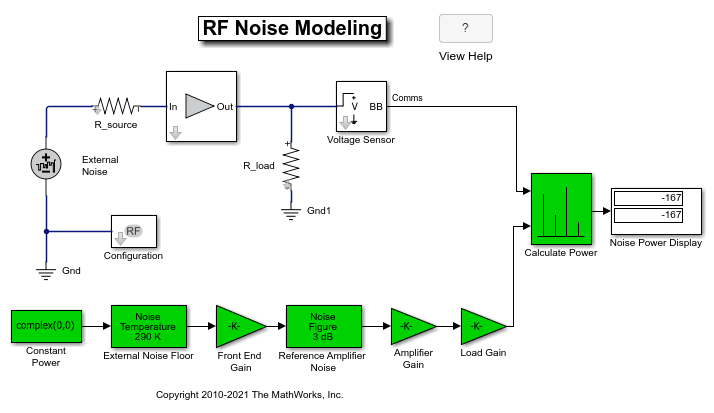## RF Noise Modeling

This example shows how to use the RF Blockset™ Circuit Envelope library to simulate noise and calculate noise power. Results are compared against theoretical calculations and a Communications Toolbox™ reference model.

The model is shown below.### System Architecture

The RF system, shown in white, consists of:

• A `Configuration` block, which sets global simulation parameters for the RF Blockset system. With the Simulate Noise option checked, noise is included in the simulation.

• An `External Noise` source with a power spectral density ofapplied at the input. In this equation,is the Boltzmann constant,is the temperature of the source, andis the noise reference impedance. The calculated noise level of -174 dBm/Hz is used in this example. The `External Noise` source is an explicit signal.

• An `Amplifier` block with a specified power gain and noise figure.

• A `Voltage Sensor` (i.e. `Outport`) block, with the Source type parameter set to Voltage.

The Communications Toolbox reference system, shown in green, consists of:

• `Gain` blocks that model amplifier gain and loading effects.

• Two `Receiver Thermal Noise` blocks that model the external noise and the amplifier noise, respectively.

The `Calculate Power` block computes RMS noise power. Note that the Communications Toolbox signal is referenced to 1 ohm, while RF Blockset power is computed for the actual load.

The example model defines variables for block parameters using a callback function. To access model callbacks, select MODELING > Model Settings > Model Properties and click the Callbacks tab in the Model Properties window.

### Running the Example

1. Type `open_system('RFNoiseExample')` at the Command Window prompt.

2. Select Simulation > Run.

The `Noise Power Display` block verifies that the RF Blockset and Communications Toolbox noise models are equivalent.

### Computing RF System Noise

To enable noise in the RF Blockset circuit envelope environment:

• In the `Configuration` block dialog, select Simulate noise.

• Specify a Temperature. RF Blockset uses this value to calculate the equivalent noise temperature inside the amplifier.

• Specify the Noise figure (dB) parameter of any amplifiers or mixers in the system.

In the example, for a specified LNA gain of 4 dB and noise figure of 3 dB, the output noise is calculated using the following equations:

``
``

The next equation converts the noise factor to an equivalent noise temperature.is the Temperature parameter of the RF Blockset `Configuration` block.

``

The final equation calculates the output noise power.is the temperature of the SimRF™ `External Noise` block and the Communications Toolbox `External Noise Floor` block.

``

The available noise power is the power that can be supplied by a resistive source when it is feeding a noiseless resistive load equal to the source resistance. The green `External Noise Floor` block generates an available power referenced to 50 ohms.

The `Front End Gain` block models the voltage divider due to the source resistance and the input impedance of the amplifier.

The green `Reference Amplifier Noise` and `Amplifier Gain` blocks model the noise added by the amplifier and the amplifier gain, respectively.

The output of the Communications Toolbox `Amplifier Gain` block is equal to the voltage across the RF Blockset `R_load` block.